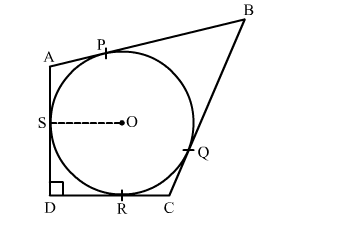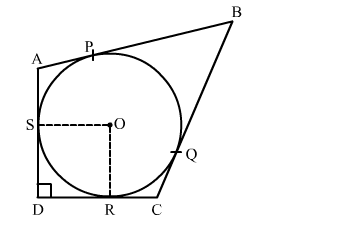# In the given figure, a circle is inscribed in a quadrilateral ABCD touching its sides Ab, BC, CD and AD at P, Q, R and S respectively.`
Question:

In the given figure, a circle is inscribed in a quadrilateral ABCD touching its sides Ab, BC, CD and AD at P, Q, R and S respectively.
If the radius of the circle is 10 cm, BC = 38 cm, PB = 27 cm and AD ⊥ CD then the length of CD is

(a) 11 cm
(b) 15 cm
(c) 20 cm
(d) 21 cmSolution:

Construction: Join ORWe know that tangent segments to a circle from the same external point are congruent.
Therefore, we have
BP = BQ = 27 cm
CQ = CR
Now, BC = 38 cm
⇒ BQ + QC = 38
⇒ QC = 38 − 27 = 11 cm
Since, all the angles in quadrilateral DROS are right angles.
Hence, DROS is a rectangle.
We know that opposite sides of rectangle are equal
∴ OS = RD = 10 cm
Now, CD = CR + RD
= CQ + RD
= 11 + 10
= 21 cm
Hence, the correct answer is option(d)